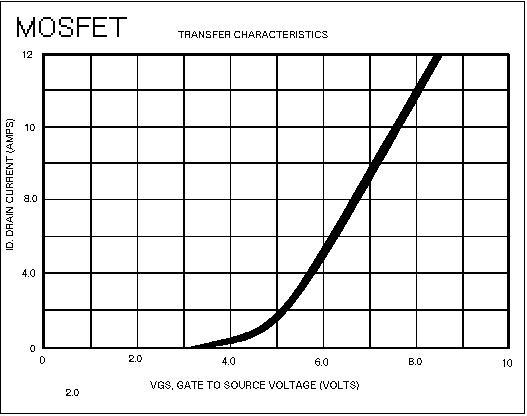# How to simplify behavior of a field-effect transistor

• SupernerdSven

#### SupernerdSven

Are there simple governing equations for FETs like there are for BJTs, and if so, what are they? For example, in the BJT case we have
IC = β×IB
IE + IC + IB

I'm sure it depends on the type - MOSFET, JFET, etc. - so anything anyone could contribute would be helpful. From what I understand, the source-to-drain current is proportional to the gate voltage, so if it's linear it would be something like
IDS = β×VG

From what I've seen Googling IV curves, that's fairly close up until saturation. Am I off base here, or am I on the right track?

Are there simple governing equations for FETs like there are for BJTs, and if so, what are they? For example, in the BJT case we have
IC = β×IB
IE + IC + IB

I'm sure it depends on the type - MOSFET, JFET, etc. - so anything anyone could contribute would be helpful. From what I understand, the source-to-drain current is proportional to the gate voltage, so if it's linear it would be something like
IDS = β×VG

From what I've seen Googling IV curves, that's fairly close up until saturation. Am I off base here, or am I on the right track?

(a) BJT
The second equation should be: IE=IB+IC.
More than that, for the BJT you forgot the control function IC=Io*[exp(VBE/Vt)-1].
This equation is important because the slope of this function gives the transconductance g=IC/Vt.

(b) FET
Here, the input-output relationship (control function) is ID=ID,max*[1-(VGS/VP)²]
with VP: pinch-off or threshold voltage, respectively.
Again, the transconductance is the slope of this function: g=2*SQRT(ID*ID,max)/|VP| .

Or you just look up the characteristics in a data sheet:the datasheet curve only works at one VDS value, usually they give you multiple curves

Thanks, everyone!
Baluncore: Wow, I must have missed that. Thanks for pointing it out!
LvW: Whoops, sorry about the typo. Yes, we only used the linear approximation in the class I took - we didn't need anything finer at the time. I appreciate your contribution!
Svein and donpacino: Thanks for pointing those out! Since I didn't feel that I knew enough about how I could use one, I hadn't looked into the datasheets much, but now I know what to expect.

the datasheet curve only works at one VDS value, usually they give you multiple curves
Yes, but when I tried to copy from a real datasheet it was "protected". So for a real datasheet, search at On Semiconductor or Texas Instruments.

Sorry, I have to correct one of my formulas (post 3). The expression in the outer brackets [... ] must be sqared:
correct: ID=ID,max*[1-(VGS/VP)]²